Power homework Help at TutorEye

## Power:

Power electronics deals in the design, development, control and transformation of power in the electrical form of energy. Power electronic system converts electrical energy into electric load through a control circuit. It involves the processing of high voltages and currents to deliver power as an output. The application of power electronics ranges from AC/DC converters, semiconductor devices to inverters.

## Power Sample Questions:

Question 1: Which of the following is true in case of forward biased p-n junction diodes?

a) The positive terminal of the battery takes electrons from the p-region
b) The positive terminal of the battery injects electrons into the p-region
c) The negative terminal of the battery takes electrons from the p-region
d) None of these

Answer: a) The positive terminal of the battery takes electrons from the p-region

Explanation: Since the diode is forward biased, the positive side is connected to p and vice versa.

Question 2: ___________ are the majority carriers in n-type material.

a) Protons            b) Electrons

c) Charges           d) None of these

Explanation: The n-region has greater concentration of electrons as compared to the p-region in a P-N junction diode.

Question 3: Find the average value of output current for a 1-phase HW diode rectifier with R load, having RMS output current of 200 A.

a)b)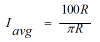c)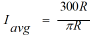d)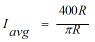Answer: d)Explanation: Given,

Question 4:  In a three phase diode half wave rectifier using 3 diodes, each diode conducts for _______ degree at a time.

a) 60                b) 120

c) 180               d) 270

Explanation: In a three phase diode half wave rectifier, only one diode conducts at a time.

Question 5: Find the average gate power dissipation when the maximum allowable gate power dissipation is 20 KW, with a duty cycle 50%.

a) 2 KW               b) 5 KW

c) 10 KW              d) 15 KW

Explanation: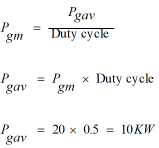Question 6: The duty cycle can be written in the form of

a) fT              b) f/T

c) T/f             d) T

Explanation:  Given, Pulse on period = T, Periodic time = f

Question 7: Find the triggering frequency when the average gate power dissipation is 0.6 W and the peak gate drive power is 10 Watts. The gate source has a pulse width of 20μsec duration.

a) 1000 Hz            b) 2000 Hz

c) 3000 Hz            d) 5000 Hz

Explanation: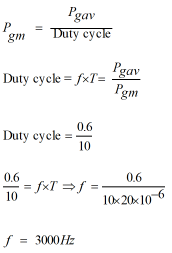Question 8: A ____________ is used to control the dc voltage to the inverter.

a) Transformer              b) Chopper

c) Converter                  d) All of the above

Explanation:  External control of dc voltage is obtained by the use of a chopper, which gets further converted from variable dc to variable ac by the converters.

Question 9: Before the invention of semiconductor technology _________ devices were used for the voltage control applications.

a) Induction machine                   b) Inverters

c) Tap changing transformer       d) None of these

Explanation:  Tap changing transformer can give variable ac from fixed ac without a change in frequency.

Question 10: ___________  controllers are used in electrical heating applications.

a) DC voltage                b) AC voltage

c) Both (a) and (b)         d) None of these

Explanation:  Variable ac supply is required for electrical heating. The devices are fired to apply enough temperature.